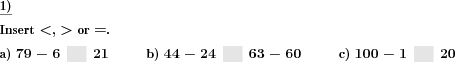Custom math worksheets at your fingertips# Details for problem "Insert relational operator for subtraction terms"

Quickname: 5303

Elementary School, Primary School.

## Summary

The correct relational operator has to be inserted for subtraction terms.

## Example## Description

In a series of problems, the correct relational operator "less than", "greater than", or "equal to" has to be chosen and inserted between to terms. One or both of these terms are either numbers or subtraction terms.

The number of problems can be selected. The number range can be chosen. Both the numbers and the sum will be within that number range.

There are options to choose from on where the subtraction terms will occur:

• random occurrence
• always on one side of the relational operator
• always on both sides of the relational operator

Download free printable worksheets for this math problem here. The worksheet contains the problems only, the solution sheet includes the answers. Just click on the respective link.

•Worksheet 1Solution sheet with answers
•Worksheet 2Solution sheet with answers
•Worksheet 3Solution sheet with answers

If you can not see the solution sheets for download, they may be filtered out by an ad blocker that you may have installed. If this is the case, please allow ads for this page and reload the page. The solution sheets will then reappear.

• Do these sample worksheets do not really fit?
• Do you need more math worksheets, with a different level of difficulty?
• Would you like to combine different problems on a worksheet and adjust them to your needs?
• As a teacher, you can put together your own worksheets using the automatically generated math problems provided.
With a free initial credit, you can start creating your own math worksheets in a few minutes.

You can try it for free! Register here, to create custom worksheets now!

## Customization options for this problem

Parameter
Possible values
Number of problems
1, 2, 3, 4, 5, 6, 7, 8, 9, 10
Number range
10, 20, 30, 40, 50, 100, 200, 500, 1000, 10000, 100000
Terms on sides
random, one side, both sides

## Similar problems

Remark
Description
The correct relational operator has to be inserted for addition terms.
Compare product terms from the times table with numbers
Compare Times table multiplication terms to numbers, decide what is the correct relational symbol.Deutsche Version dieser Aufgabe
These informational pages with samples describe math problems that can be combined on custom math worksheets with solutions for home and K-12 school use.
Deutsche Seiten
×